Conversions and Transformations using the Solid Edge API – Part 4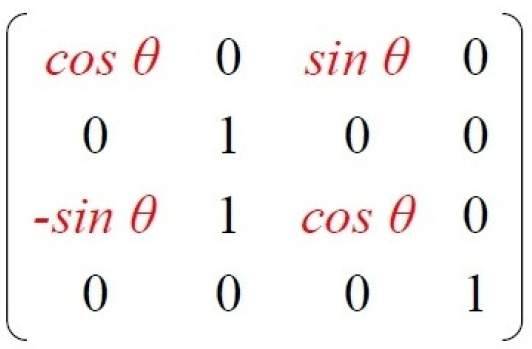…continued from part 3

This series of articles discusses the following:

1. Document Unit Conversion – ParseUnit and Format Unit methods of the UnitsOfMeasure object.

2. View Coordinate Conversion – ViewToSheetViewToSheetViewToSheetViewToSheetViewToSheet and SheetToView methods of the DrawingView object.

3. 2D to 3D Conversion – Convert2DCoordinate method of the Profile object.

4. 3D to 2D Transformations – ModelToView method of the DrawingView object.

5. Assembly Transformations – GetMatrix and PutMatrix methods of the Occurrence object.

Assembly Transformations

This part of the conversion and transformation series discusses the transformation of occurrences in the assembly using matrices. These transformations are movement and rotation.

An Occurrence object in the assembly has a Matrix Property which is an array and can be accessed as below:

Imports SolidEdgeAssembly

Module Module1

Sub Main()
Dim seApp As SolidEdgeFramework.Application = Runtime.InteropServices.Marshal.GetActiveObject(“SolidEdge.Application”)
Dim seDoc As AssemblyDocument = seApp.ActiveDocument
Dim seOccs As Occurrences = seDoc.Occurrences
Dim seOcc As Occurrence = seOccs.Item(1)

Dim Matrix = Array.CreateInstance(GetType(Double), 16)
seOcc.GetMatrix(Matrix)

Debug.Print(Matrix.GetValue(0))
.
.
.
Debug.Print(Matrix.GetValue(15))

Note the array of size 16 and index from 0 to 15 which can be represented in a matrix form as shown below: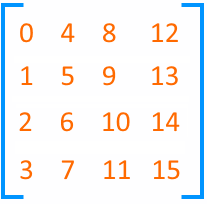The position of each element has a special meaning in the matrix.

For example, the positions highlighted below are the translations, meaning moving an occurrence along the X, Y and Z directions respectively.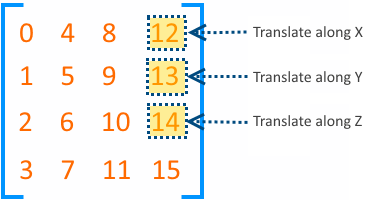These correspond to the elements at position 12, 13 and 14 in the array.

Therefore, to move an occurrence along the X-Axis:

1. Get the occurrence matrix into an array of Doubles.

2. Change the 12th element in the array.

3. Assign the matrix back to the occurrence.

seMatrix.SetValue(0.125, 12)
seOcc.PutMatrix(seMatrix, True)
End Sub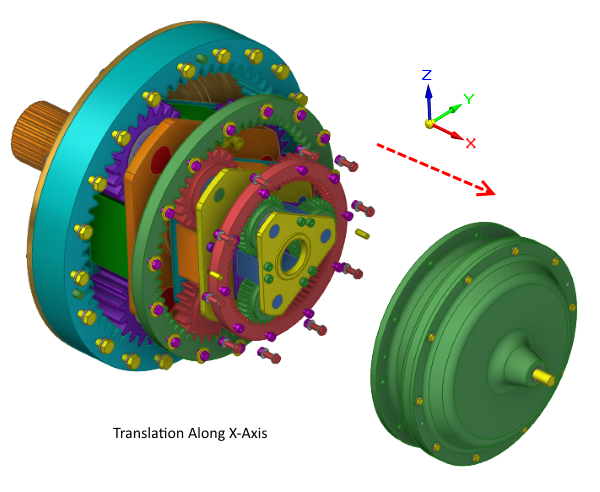Similarly, to rotate the occurrence about the Z-axis by 30, the calculations are as shown in the image below and assign to the corresponding 4 elements of the matrix.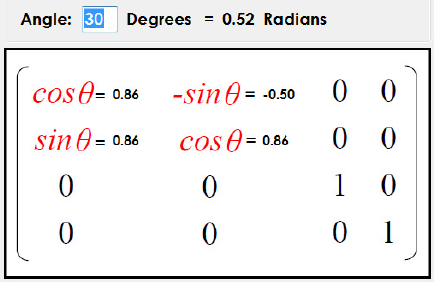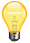Note: The rotation angle in radians array:

To rotate an occurrence about the X-axis: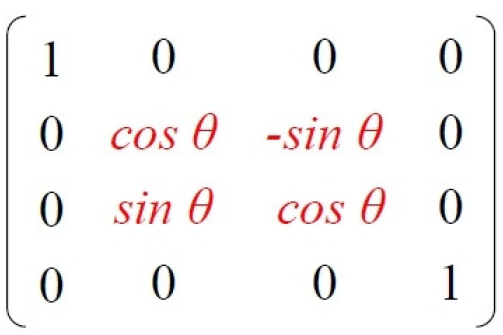To rotate an occurrence about the Y-axis:This concludes the series on Conversion and Transformations using the Solid Edge API.

If you have any questions, please use the Solid Edge Developer forum to post messages.